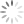Loading, please wait...Showing articles with Data Structure Tutorial. Show all articles
In this article, you will understand the Fibonacci operations of the Decrease key and delete the node.
Data Structure tutorialIn this article, you will understand the Depth First Search and Breadth First Search algorithm in data structure
In this article, you will understand the Fibonacci Heap Data Structure in better detail.
In this article, you will understand the Heap Sort Algorithm of the Data Structure in better detail.
In this article, you will understand the Bucket Sort Algorithm of the Data Structure in better detail.
In this article, you will learn about the insertion sort algorithm in the data structure in brief.
In this article, you will understand the bubble sort in the data structure in brief.
In this article, you will understand how to perform deletion from a Red-Black Tree.
In this article, you will understand the two operations on a B+ tree i.e insertion and deletion.
In this article, you will understand the Complete and Balanced binary tree.
In this article, you will understand the Full and Perfect Binary Tree in a binary tree.
In this article, you will understand different types of tree traversal techniques.
In this article, you will understand what binary trees are and their different types.
In this article, you will learn about the Tree Data Structure and other different types of trees.
In this article, you will understand what is the Decrease key and Delete node operations on a Fibonacci Heap.
In this article, you will learn the following concepts: What is Fibonacci Heap? What are the Properties of a Fibonacci Heap? How to represent the memory of the Node in a Fibonacci Heap? What Operations can be done on a Fibonacci Heap?